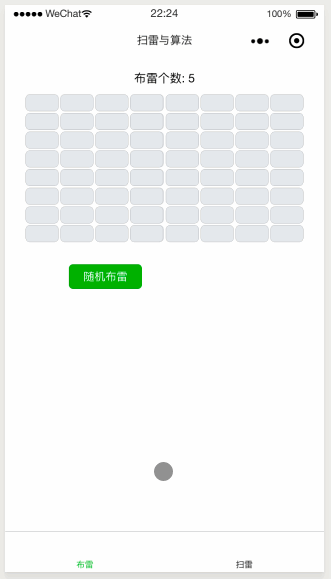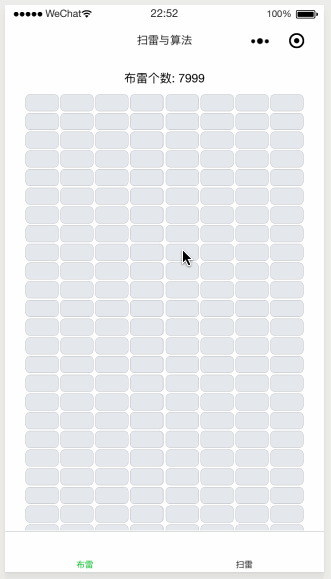5

### 方法一

for (var i = 0; i < mineNumber; i++) {       var row = this.rangeRandom(0, this.rowCount - 1);       var col = this.rangeRandom(0, this.colCount - 1);       //使用数字 9 表示该区域有雷       tmpMineMap[row][col] = 9; }### 方法二

• 如果该位置是空的，那么则布雷，然后进行寻找新的位置布下下一颗雷

• 如果该位置已经被安置了雷，那么就需要重新生成一个位置来安置

   for (var i = 0; i < mineNumber; i++) {      //通过死循环来实现不停的寻找，直到安置好雷      while (true) {        var row = this.rangeRandom(0, this.rowCount - 1);        var col = this.rangeRandom(0, this.colCount - 1);        //用数字 9 表示该区域有雷，如果该位置没有布雷，那么则放置        if (tmpMineMap[row][col] != 9) {           tmpMineMap[row][col] = 9;           //跳出循环           break;        }      }    }### 方法三

//先按顺序排列for (var i = 0; i < mineNumber; i++) {    var row = parseInt(i / this.colCount);    var col = i % this.colCount;    //使用数字 9 表示该区域有雷    tmpMineMap[row][col] = 9;}//定义交换的次数，次数越多越混乱随机var swapTime = 100;for (var i = 0; i < swapTime; i++) {    //随机位置1    var row1 = this.rangeRandom(0, this.rowCount - 1);    var col1 = this.rangeRandom(0, this.colCount - 1);    //随机位置2    var row2 = this.rangeRandom(0, this.rowCount - 1);    var col2 = this.rangeRandom(0, this.colCount - 1);    //交换两个位置    var temp = tmpMineMap[row1][col1];    tmpMineMap[row1][col1] = tmpMineMap[row2][col2];    tmpMineMap[row2][col2] = temp;}

### 总结